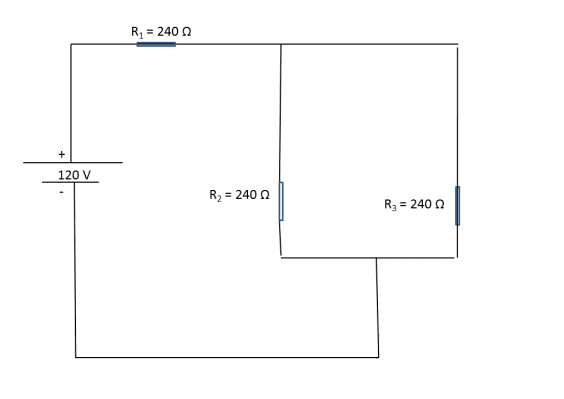# Power Loss, Current, Resistors, and Voltage

Jakarto

## Homework Statement

Consider the circuit shown below. a) What is the total power loss for the whole circuit? b) What is the power loss through R1? c) What is the power loss through R2? d) Find the voltage across each of the three resistors.

I didn't do this myself. I have no idea what's going on, if these answers are correct, it's because someone answered them for me on Chegg, otherwise I have no idea. Please give me the best help you guys can.
[/B]## Homework Equations

Rnet = (R2 * R3) / (R2 + R3) +(R1)
I = V / Rnet
Ptotal_loss = VI
PR1 = I^2 * R1
PR2 = I'^2 * R2 ? what is I'^2?[/B]

## The Attempt at a Solution

Rnet = (240*240)ohm / (240+240)ohm +(240 ohm) = 360 ohm.

I = (120V) / (360 ohm) = 0.333A

a) Ptotal_loss = 120V * 0.333A = 40W

b) PR1 = (0.333A^2) * (240 ohm) = 26.61W

c) PR2 = I'^2 * R2 = I'^2 = I'^2 * (240 ohm) = 6.68W? How, why and what is I'^2? Is this answer even correct?

d) So, the potential drops, and the answer is R1 = 80V apparently and
R2 = R3 = 40V? How? What formula(s)? All I know is V = IR, but it is not working here. [/B]

Passionate Eng
check V=IR again
it works

Jakarto
can you show me, because see look:
V = 0.333A * 120 = 40V, but what about for R1? How is the answer to that 80V?
Also, how is PR2 = 6.68W. Can you give me the appropriate formula for that? I cannot figure it out.

Homework Helper
Gold Member

Rnet = (240*240)ohm / (240+240)ohm +(240 ohm) = 360 ohm.
That's correct but with experience you will recognise that if two identical resistors are in parallel then the equivalent resistance is half so in this case I would write this (where "//" means "in parallel")...

Req = R2//R3 + R1
R2//R3 = 240//240 = 120
R1 = 240
so
Req = 120 + 240 = 360 Ohms

Then you/they correctly calculate the current flowing from the battery..
I = (120V) / (360 ohm) = 0.333A
and the total power delivered by the battery (which by CoE is the same as that dissipated in the rest of the circuit)...
a) Ptotal_loss = 120V * 0.333A = 40W
All of the current flows through R1 so calculating the power in R1 is easy..
Using V=IR and P=IV you can write P=I2R1 and so...
b) PR1 = (0.333A^2) * (240 ohm) = 26.61W
Then calculate the power in R2...
c) PR2 = I'^2 * R2 = I'^2 = I'^2 * (240 ohm) = 6.68W? How, why and what is I'^2? Is this answer even correct?
Yes it's correct. However it needs explanation...
Because R2=R3 the current in R1 is split equally between R2 and R3. So the current flowing in R2 = 0.3333/2 = 0.16666 A.
Then applying PR2=I2R2 = 0.16662 * 240 = 6.667 W
d) So, the potential drops, and the answer is R1 = 80V apparently
Correct. The potential drop across R1 is found using V=IR = 0.333*240 = 80V
R2 = R3 = 40V? How?

There three different ways to calculate the voltage drop across R2 and R3...

1) The battery is 120V. Then 80V is "lost" across R1 leaving 120-80=40V across R2 and R3. This is applying kirchhoff's voltage law (google it) that says the sum of voltages in a loop is zero (in this case +120-80-40=0).

2) Using V=IR. We calculated the current flowing in R2 in Qc as 0.16666 A so using V=IR...
V = 0.1666 * 240 = 40V

3) The potential divider method (google it)...

V = 120 * (R2//R3) / (R1 + R2//R3)
= 120 * 120 / (360)
= 40V

PS: Sorry I've been a bit inconsistent with the number of decimal places I've used.

•Jakarto
Jakarto
Thank You so much CWaters!